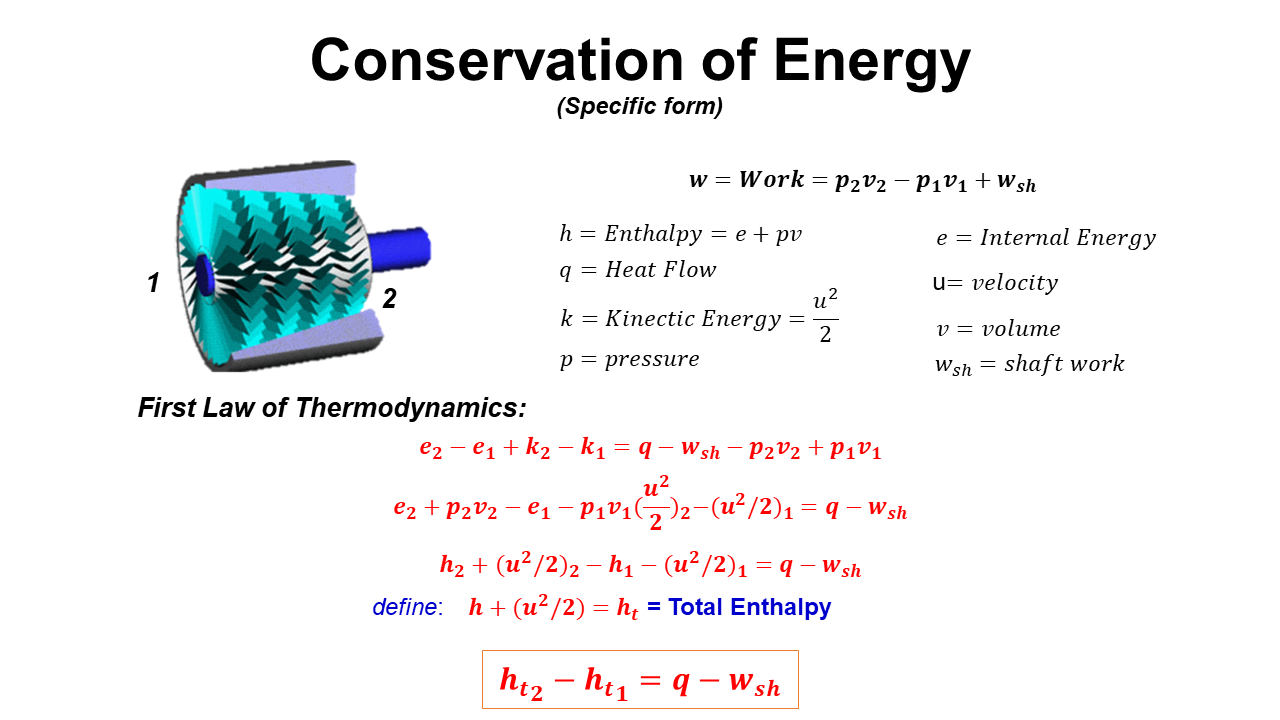# Conservation of EnergyThe conservation of energy is a fundamental concept of physics along with the conservation of mass and the conservation of momentum. Within some problem domain, the amount of energy remains constant, and energy is neither created nor destroyed. Energy can be converted from one form to another (potential energy can be converted to kinetic energy) but the total energy within the domain remains fixed.

## Derivation

On some separate pages, we have discussed the state of a static gas, the properties which define the state, and the first law of thermodynamics as applied to any system, in general. On this page we derive a useful form of the energy conservation equation for a gas beginning with the first law of thermodynamics. If we call the internal energy of a gas E, the work done by the gas W, and the heat transferred into the gas Q, then the first law of thermodynamics indicates that between state “1” and state “2”:

#### E2 – E1 = Q – W

Aerospace engineers usually simplify a thermodynamic analysis by using intensive variables; variables that do not depend on the mass of the gas. We call these variables specific variables. We create a “specific” variable by taking a property whose value depends on the mass of the system and dividing it by the mass of the system. Many of the state properties listed on this page, such as the work and internal energy depend on the total mass of gas. We will use “specific” versions of these variables. Engineers usually use the lower-case letter for the “specific” version of a variable.

Our first law equation then becomes:

#### e2 – e1 = q – w

Because we are considering a moving gas, we add the specific kinetic energy term to the internal energy on the left side. The normal kinetic energy K of a moving substance is equal to 1/2 times the mass m times the velocity u squared:

#### K = (m * u^2) / 2

Then the specific kinetic energy k is given by:

#### k = (u^2) / 2

and the first law equation becomes:

## Shaft Work

There are two parts to the specific work for a moving gas. Some of the work, called the shaft work (wsh) is used to move the fluid or turn a shaft, while the rest of the work goes into changing the state of the gas. For a pressure p and specific volume v, the work is given by:

Substituting:

#### e2 – e1 + k2 – k1 = q – (p * v)2 + (p * v)1 – wsh

If we perform a little algebra on the first law of thermodynamics, we can begin to group some terms of the equations. :

#### e2 + (p * v)2 – e1 – (p * v)1 + [(u^2) / 2]2 – [(u^2) / 2]1 = q – wsh

A useful additional state variable for a gas is the specific enthalpy h which is equal to:

#### h = e + (p * v)

Simplifying the energy equation:

or

#### h2 + [(u^2) / 2]2 – h1 – [(u^2) / 2]1 = q – wsh

By combining the velocity terms with the enthalpy terms to form the total specific enthalpy “ht” we can further simplify the equation.

#### ht = h + u^2 / 2

The total specific enthalpy is analogous to the total pressure in Bernoulli’s equation; both expressions involve a “static” value plus one half the square of the velocity.

The final, most useful, form of the energy equation is given in the red box.

#### ht2 – ht1 = q – wsh

For a compressor or power turbine, there is no external heat flow into the gas and the “q” term is set equal to zero. In the burner, no work is performed and the “wsh” term is set to zero.

Provide feedback